## Mensuration

### Suggested Lessons

1. Triangles: A triange is a polygon having three sides and three angles. i. Sum of the angles of a triangle is 180°.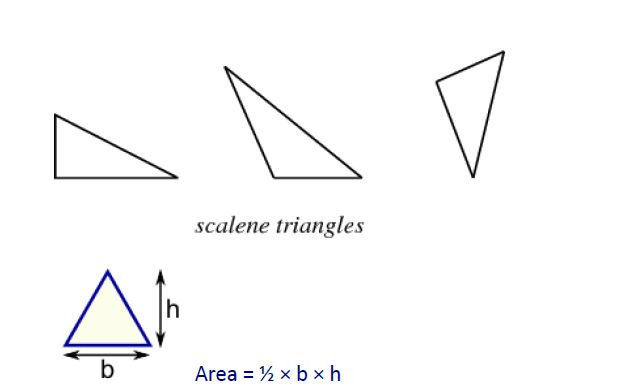Where b = base and h = vertical height Perimeter is sum of three sides. The sum of any two sides of a triangle is greater than the third side. Area = $$\frac {1}{2}$$x Base x Height. = $$\frac {1}{2}$$b x h Area of a triangle = s(s-a)(s-b)(s-c) where a, b, c are the sides of the triangle and s =$$\frac {1}{2}$$(a + b + c). ii. Pythagoras Theorem: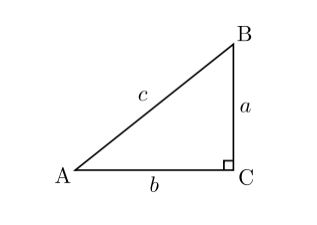In

a right-angled triangle, (Hypotenuse)2 =+. In above diagram angle C is right angle i.e 90°=+=iii. The line joining the mid-point of a side of a triangle to the positive vertex is called the median. iv. The point where the three medians of a triangle meet, is called centroid. The centroid divided each of the medians in the ratio 2 : 1. v. In an isosceles triangle, the altitude from the vertex bisects the base.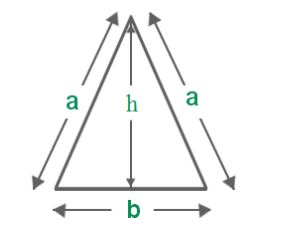Perimeter = a + a + b = 2a + b Area = $$\frac {1}{2}$$x Base x Height. =$$\frac {1}{2}$$b x h Here=- $$\frac {b}{2}$$vi. The median of a tri angle divides it into two triangles of the same area. vii. The area of the triangle formed by joining the mid-points of the sides of a given triangle is one-fourth of the area of the given triangle.

### Equilateral triangle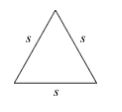Three sides are equal and three angles are equal. If s is the side of an equilateral triangle then Perimeter = 3 s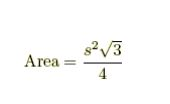Quadrilateral is a four-sided figure. May be a rectangle, Square, Parallelogram, a Rhombus or a trapezium.

### Rectangle-

A rectangle is a quadrilateral with four right angles. It can also be defined as an equiangular quadrilateral, since equiangular means that all of its angles are equal. If two opposite sides of a rectangle are w and h then perimeter Perimeter of a rectangle = 2(Length + Breadth) = 2(w + h) Area of a rectangle = (Length x Breadth). Area = w x h And diagonals of a rectangle are equal and bisect each other.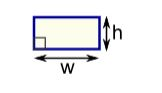### Square-

A square is a four sided figure created by connecting four line segments. All of the line segments are of the same length and they come together to form four right angles. All four sides are equal and four angles are equal = 90 degree. If a is each side of a square then Perimeter = 4 a And area of a square A = aThe diagonals of a square are equal and bisect each other at right angles.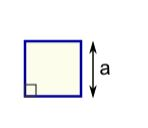### Rhombus-

A rhombus is a four-sided shape where all sides have equal length. Also opposite sides are parallel and opposite angles are equal. Another interesting thing is that the diagonals (dashed lines in second figure) meet in the middle at a right angle. In other words they "bisect" (cut in half) each other at right angles.A rhombus is a special type of parallelogram of four equal sides. The diagonals of a rhombus are unequal and bisect each other at right angles.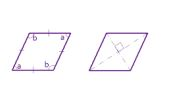The area is the product of two adjacent sides. i.e ba b is the length of the base a is the altitude (height). Another simple formula for the area of a rhombus when we know the lengths of the diagonals. The area is half the product of the diagonals. As a formula:

Area  $$\frac {b}{2}$$d1 x d2

Where d1 is the length of a diagonal d2 is the length of the other diagonal

### Parallelogram

A parallelogram is a four-sided plane rectilinear figure with opposite sides parallel. A parallelogram and a rectangle on the same base and between the same parallels are equal in area. Area of parallelogram = (Base x Height).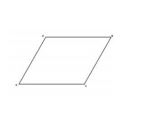### Trapezeom-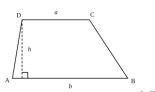A trapezium is a quadrilateral with one pair of sides parallel. Area of a trapezium = $$\frac {b}{2}$$x (sum of parallel sides) x distance between them. Area = $$\frac {b}{2}$$h (a +b )